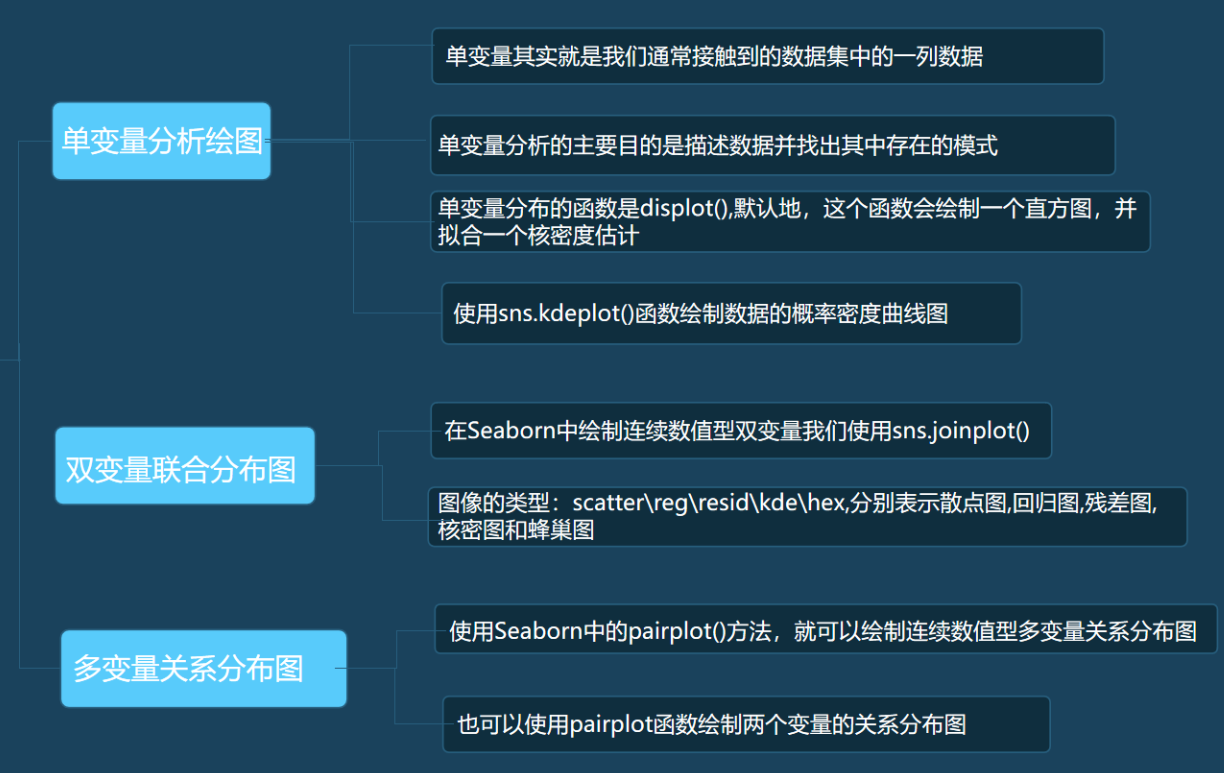# python数据分析010_seaborn的绘图方式

1.什么是单变量分析？

单变量其实就是我们通常接触到的数据集中的一列数据

2.使用NumPy模块从标准正态分布中随机地抽取1000个数，作为我们的连续数值型数据。

data = np.random.normal(size=1000) random是NumPy的一个随机模块，在random模块中的normal方法表示从正态分布中随机产生size个数值。

3.在seaborn里最常用的观察单变量分布的函数是distplot()，默认地，这个函数会绘制一个直方图，并拟合一个核密度估计。

sns.distplot(data, bins, hist = True, kde = True)

data参数记录绘图所用的数据，而bins参数在绘制直方图时可以进行设置，用于设置分组的个数，默认值时，会根据数据的情况自动分为n个组，若是想指定分组的个数，可以设置该参数，然后计算我们可以增加其数量，来看到更为详细的信息。

hist和kde参数用于调节是否显示直方图及核密度估计图，默认hist、kde均为True，表示两者都显示。我们可以通过修改参数为False选择是否将其中之一去掉。

• import numpy as np
• import seaborn as sns
• # 从标准正态分布中随机地抽取1000个数
• data = np.random.normal(size=1000)
• sns.set(style='darkgrid')
• sns.distplot(data,10,kde=True, hist=True)

4.使用sns.kdeplot()函数绘制数据的概率密度曲线图。

• import numpy as np
• import seaborn as sns
• data = np.random.normal(size=1000)
• sns.set(style='darkgrid')

1.在Seaborn中绘制连续数值型双变量我们使用sns.jointplot():

seaborn.jointplot(x, y, data=None, kind='scatter')

x、y：分别记录x轴和y轴的数据名称。

data：数据集，data的数据类型为DataFrame。

kind：用于设置图像的类型，可选的类型有：'scatter' | 'reg' | 'resid' | 'kde' | 'hex'，分别表示散点图、回归图、残差图、核密度图和蜂巢图。
• import numpy as np
• import pandas as pd
• import matplotlib.pyplot as plt
• import seaborn as sns
• # 创建dataframe: df
• df = pd.DataFrame({'x': np.random.normal(size=500),
• 'y': np.random.normal(size=500)})
• # 绘制双变量散点图
• sns.jointplot(x='x', y='y', data=df,kind='reg')
• plt.show()

1.使用seaborn中的pairplot()方法，就可以绘制连续数值型多变量关系分布图

sns.pairplot( data, hue, vars, kind, diag_kind)

参数介绍

• data表示绘图所用到的数据集
• hue参数表示按照某个字段进行分类
• vars参数可以用于筛选绘制图像的变量，用列表的形式传入列名称
• kind参数用于设置变量间图像的类型，可以选择'scatter'散点图，或者 'reg'回归图
• diag_kind用于设置对角线上的图像类型，可以选择'hist'直方图， 或者'kde'核密度图

代码:

• import pandas as pd
• import matplotlib.pyplot as plt
• import seaborn as sns